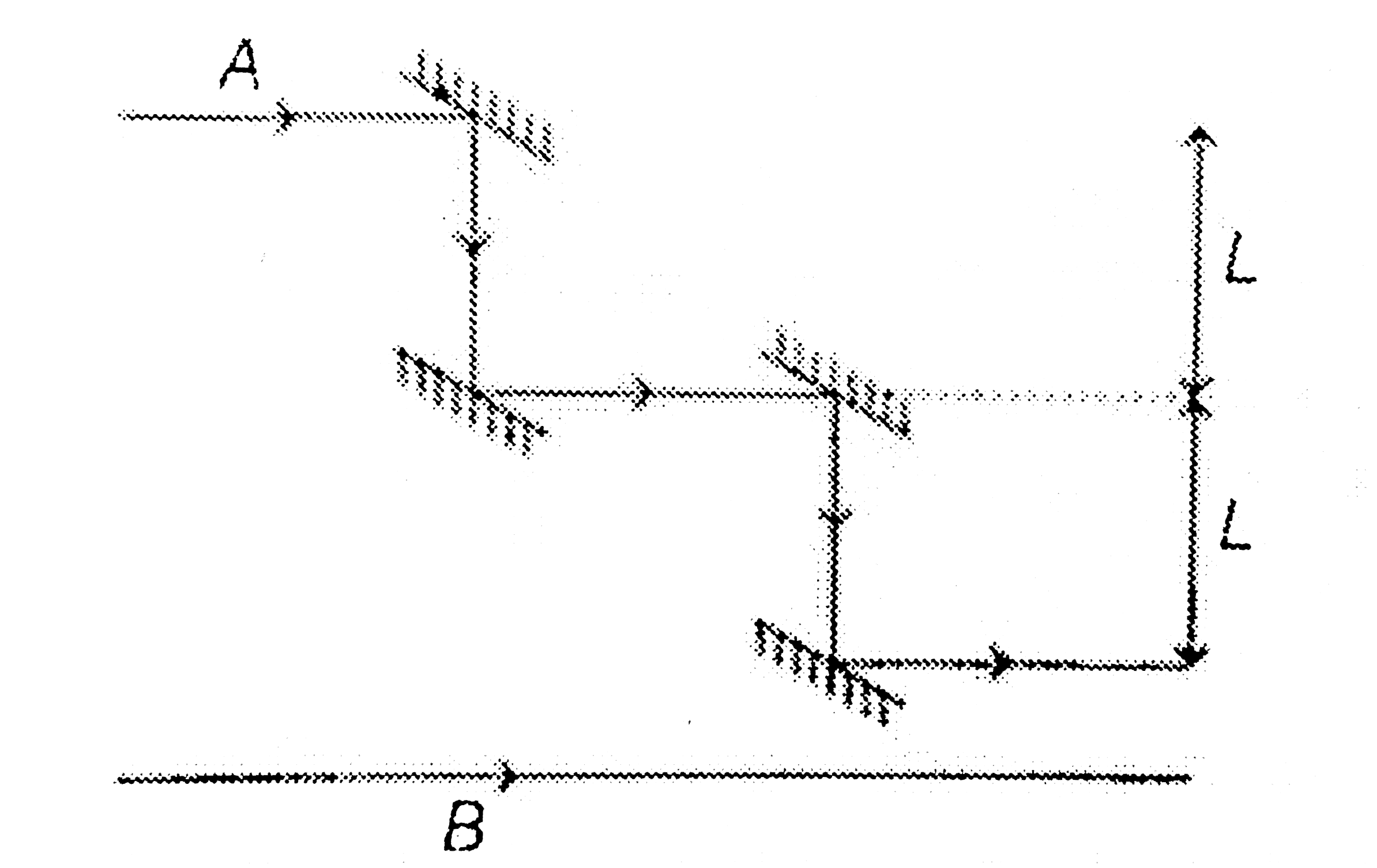# In the figure shown, light waves A and B both of wavelength lambda are initially in phase and travelling rightward as incident by the two rays. Wave

14 views
in Physics
In the figure shown, light waves A and B both of wavelength lambda are initially in phase and travelling rightward as incident by the two rays. Wave A is reflected from four parallel surfaces but ends up travelling in original direction. The possible value(s) of distance L in terms of wavelength lambda in figure which put A and B exactly out of phase with each other after all the reflected is/areA. lambda//2
B. 3lambda//2
C. 3lambda//4
D. lambda

by (73.0k points)